﻿ 仿生粘附式尺蠖机器人设计及分析 The Design and Analysis of the Biomimetic Adherent Inchworm-Like Robot

Journal of Aerospace Science and Technology
Vol.05 No.04(2017), Article ID:23218,18 pages
10.12677/JAST.2017.54022

The Design and Analysis of the Biomimetic Adherent Inchworm-Like Robot

Yanmao Ye1, Yaolei Zhang2, Hu Huang2, Guochen Fan2, Mingying Huo1*, Naiming Qi1

1Harbin Institute of Technology, Harbin Heilongjiang

2China Academy of Launch Vehicle Technology, Beijing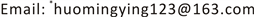Received: Dec. 7th, 2017; accepted: Dec. 21st, 2017; published: Dec. 28th, 2017ABSTRACT

In view of the need of future space weapons’ development, it proposes a kind of biomimetic adherent inchworm-like robot, which combines the biomimetic gecko adhesive material and the movement mode of geometer. The mechanism, electrical system and control strategy of the biomimetic adherent inchworm-like robot are designed in this paper. Then, it designs the interface relationships between each electrical element and the semi-autonomous control strategy. The major structure and adherent material structure of adherent sucker are designed in this paper. Then it accomplishes the comparison of the adherent material and the calculation of the adhesive power. After that, it designs three range sensors for the adherent sucker. And base on the range sensors, it accomplishes the calculation of the position and pose between the adherent sucker and target surface. This paper uses the Deanvit-Hartenberg method to set up the kinematic model of the biomimetic adherent inchworm-like robot. Then it accomplishes the gait planning of the robot, and designs the “One Gait”. At last, it sets up the virtual prototype model of the robot in the software Adams, and it carries out the simulation of robot’s gait, verifying the correctness of the gai planning.

Keywords:Space Weapon, Biomimetic, Inchworm-Like Robot, Mechanism Modeling, Kinematics, Gait Planning

1哈尔滨工业大学，航空宇航与力学工程系，黑龙江 哈尔滨

2中国运载火箭技术研究院，北京Copyright © 2017 by authors and Hans Publishers Inc.

This work is licensed under the Creative Commons Attribution International License (CC BY).

http://creativecommons.org/licenses/by/4.0/1. 引言

2. 仿生黏附式尺蠖机器人设计

2.1. 仿生粘附式尺蠖机器人机构设计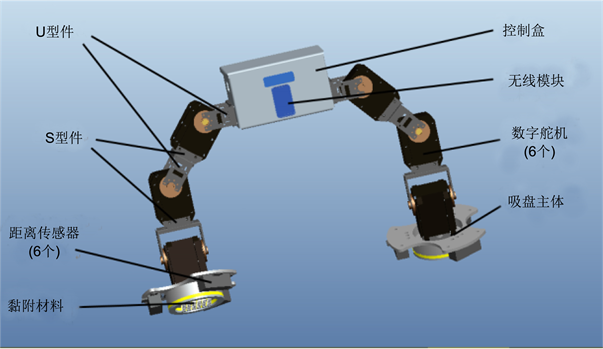Figure 1. General design structure of the biomimetic adherent inchworm-like robot

2.2. 仿生粘附式子尺蠖机器人控制策略设计

1) 6个距离传感器测得吸盘上三个点与目标平面的距离，6个舵机的绝对码盘测得舵机轴的绝对转角，传送到控制器。

2) 控制器根据距离传感器的数值解算吸盘底部平面与目标平面的姿态和距离关系，根据舵机轴的转角解算尺蠖机器人的姿态。之后根据吸盘与目标平面的关系进行机器人步态的规划，计算出6个舵机和粘附吸盘的控制量，并输送给执行机构。

3) 执行机构接收到控制器的控制指令后，驱动6舵机转动到对应的角度，并控制吸盘的通断状态。

4) 尺蠖机器人完成一个步态，进入下一个步态。Figure 2. Practicality picture of the biomimetic adherent inchworm-like robot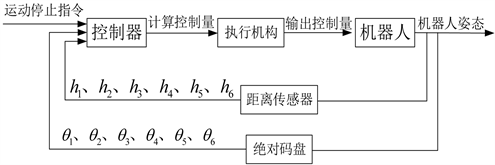Figure 3. Conceptual sketch of control strategy

3. 仿生粘附式尺蠖机器人粘附吸盘设计

3.1. 粘附吸盘结构详细设计

1) 粘附材料的结构设计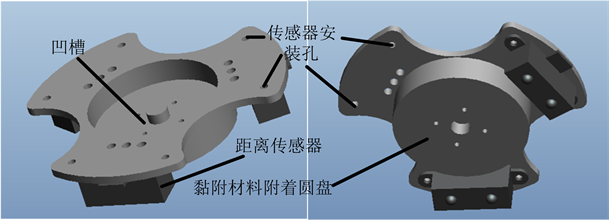Figure 4. Conceptual sketch of control strategyFigure 5. Structure drawing of the adherent material

2) 粘附材料的选型

3.2. 吸盘底部与目标平面的位姿解算

3.2.1. 目标平面姿态的解算Table 1. Comparison table of different gecko-seta-like dry adhesive material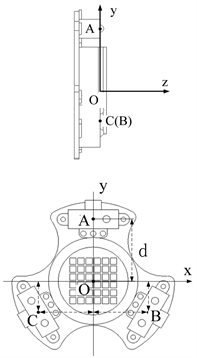Figure 6. Conceptual sketch of the distance sensor’s coordinate

${A}^{\prime }$${B}^{\prime }$${C}^{\prime }$ 所在的目标平面的平面方程为：

$x+ay+bz+c=0$

${A}^{\prime }$${B}^{\prime }$${C}^{\prime }$ 的坐标代入平面方程中，可以解得：

$\left\{\begin{array}{l}a=\frac{2\left({h}_{1}-{h}_{2}\right)\left({h}_{2}-{h}_{3}\right)}{3\sqrt{3}{d}^{2}}+\frac{\sqrt{3}}{3}\\ b=\frac{{h}_{3}-{h}_{2}}{\sqrt{3}d}\\ c=\frac{2\left({h}_{2}-{h}_{1}\right)\left({h}_{2}-{h}_{3}\right)+3{h}_{1}\left({h}_{2}-{h}_{3}\right)}{3\sqrt{3}d}-\frac{\sqrt{3}}{3}d\end{array}$

3.2.2. 目标平面与吸盘距离的解算Figure 7. Position sketch of the points in distance sensor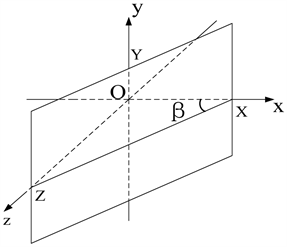Figure 8. Position relationship of target plane and coordinate after first rotation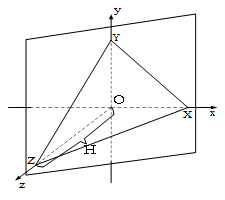Figure 9. Distance sketch of the target plane and sucker

4. 仿生粘附式尺蠖机器人运动学建模

4.1. 坐标系建立

4.2. 运动学方程推导

4.2.1. D-H参数的建立

4.2.2. 仿生粘附式尺蠖机器人的正运动学

$\begin{array}{l}{}^{i-1}A{}_{i}=Tran{s}_{z}\left({d}_{i}\right)Ro{t}_{z}\left({\theta }_{i}\right)Tran{s}_{x}\left({a}_{i}\right)Ro{t}_{x}\left({\alpha }_{i}\right)\\ =\left[\begin{array}{cccc}\mathrm{cos}{\theta }_{i}& -\mathrm{cos}{\alpha }_{i}\mathrm{sin}{\theta }_{i}& \mathrm{sin}{\alpha }_{i}\mathrm{sin}{\theta }_{i}& {a}_{i}\mathrm{cos}{\theta }_{i}\\ \mathrm{sin}{\theta }_{i}& \mathrm{cos}{\alpha }_{i}\mathrm{cos}{\theta }_{i}& -\mathrm{sin}{\alpha }_{i}\mathrm{cos}{\theta }_{i}& {a}_{i}\mathrm{sin}{\theta }_{i}\\ 0& \mathrm{sin}{\alpha }_{i}& \mathrm{cos}{\alpha }_{i}& {d}_{i}\\ 0& 0& 0& 1\end{array}\right]\end{array}$ (4-1)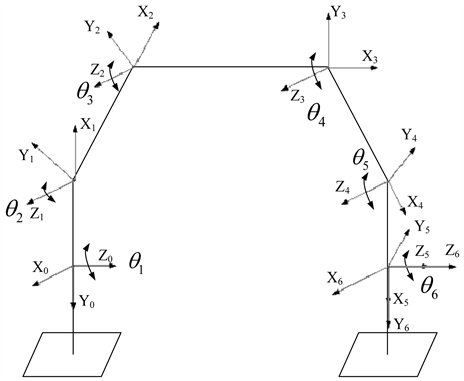Figure 10. The coordinate of inchworm-like robotTable 2. D-H parameter table of inchworm-like robot

$\begin{array}{c}{}^{0}A{}_{6}={}^{0}A{}_{1}{}^{1}A{}_{2}{}^{2}A{}_{3}{}^{3}A{}_{4}{}^{4}A{}_{5}{}^{5}A{}_{6}\\ =\left[\begin{array}{l}{s}_{1}{s}_{6}+{c}_{1}{c}_{6}{c}_{2345}\text{ }\text{\hspace{0.17em}}{s}_{1}{c}_{6}-{c}_{1}{s}_{6}{c}_{2345}\\ -{c}_{1}{s}_{6}+{s}_{1}{c}_{6}{c}_{2345}\text{\hspace{0.17em}}\text{\hspace{0.17em}}-{c}_{1}{c}_{6}-{s}_{1}{s}_{6}{c}_{2345}\\ -{c}_{6}{s}_{2345}\text{ }\text{ }\text{ }\text{ }{s}_{6}{s}_{2345}\\ 0\text{ }\text{ }\text{ }\text{ }\text{ }\text{ }\text{\hspace{0.17em}}\text{\hspace{0.17em}}\text{\hspace{0.17em}}0\text{ }\text{ }\text{ }\text{ }\text{\hspace{0.17em}}0\end{array}\\ \text{\hspace{0.17em}}\text{\hspace{0.17em}}\begin{array}{l}-{c}_{1}{s}_{2345}\text{\hspace{0.17em}}\text{\hspace{0.17em}}{a}_{1}{c}_{1}+{a}_{2}{c}_{1}{c}_{2}+{a}_{3}{c}_{1}{c}_{23}+{a}_{4}{c}_{1}{c}_{234}+{a}_{5}{c}_{1}{c}_{2345}\\ {a}_{1}{s}_{1}+{a}_{2}{s}_{1}{c}_{2}+{a}_{3}{s}_{1}{c}_{23}+{a}_{4}{s}_{1}{c}_{234}+{a}_{5}{s}_{1}{c}_{2345}\\ -{a}_{2}{s}_{2}-{a}_{3}{s}_{23}-{a}_{4}{s}_{234}-{a}_{5}{s}_{2345}\\ 1\end{array}\right]\end{array}$ (4-2)

4.2.3. 仿生粘附式尺蠖机器人的逆运动学

$\begin{array}{c}{q}_{1}={\theta }_{1}=\mathrm{arctan}2\left(\frac{-{\stackrel{^}{z}}_{1}}{{\stackrel{^}{z}}_{2}}\right)±\mathrm{arctan}2\left(\frac{\sqrt{{\left(-{\stackrel{^}{z}}_{1}\right)}^{2}+{\stackrel{^}{z}}_{2}^{2}-0}}{0}\right)\\ =\mathrm{arctan}2\left(\frac{-{\stackrel{^}{z}}_{1}}{{\stackrel{^}{z}}_{2}}\right)±\frac{\text{π}}{2}\end{array}$ (4-3)

${q}_{6}={\theta }_{6}=\mathrm{arctan}2\left(\frac{{\stackrel{^}{x}}_{2}\mathrm{cos}{\theta }_{1}-{\stackrel{^}{x}}_{1}\mathrm{sin}{\theta }_{1}}{{\stackrel{^}{y}}_{2}\mathrm{cos}{\theta }_{1}-{\stackrel{^}{y}}_{1}\mathrm{sin}{\theta }_{1}}\right)$ (4-4)

${q}_{3}={\theta }_{3}=\mathrm{arctan}2\left(\frac{{K}_{1}}{{K}_{2}}\right)±\mathrm{arctan}2\left(\frac{\sqrt{{K}_{1}^{2}+{K}_{2}^{2}-{K}_{3}^{2}}}{{K}_{3}}\right)$ (4-5)

${K}_{1}=-2{a}_{2}{a}_{4}\mathrm{sin}{\theta }_{4}$ , ${K}_{2}=2{a}_{2}\left({a}_{3}+{a}_{4}\mathrm{cos}{\theta }_{4}\right)$ , ${K}_{3}={A}^{2}+{B}^{2}-{a}_{2}^{2}-{\left({a}_{3}+{a}_{4}\mathrm{cos}{\theta }_{4}\right)}^{2}-{a}_{4}^{2}\mathrm{sin}{\theta }_{4}^{2}$

$\begin{array}{l}A={a}_{2}\mathrm{cos}{\theta }_{2}+{a}_{3}\mathrm{cos}\left({\theta }_{2}+{\theta }_{3}\right)+{a}_{4}\mathrm{cos}\left({\theta }_{2}+{\theta }_{3}\right)\mathrm{cos}{\theta }_{4}\\ \text{\hspace{0.17em}}\text{ }\text{\hspace{0.17em}}\text{ }-{a}_{4}\mathrm{sin}\left({\theta }_{2}+{\theta }_{3}\right)\mathrm{sin}{\theta }_{4}\\ \text{ }={a}_{2}\mathrm{cos}{\theta }_{2}+\left({a}_{3}+{a}_{4}\mathrm{cos}{\theta }_{4}\right)\mathrm{cos}\left({\theta }_{2}+{\theta }_{3}\right)\\ \text{ }\text{\hspace{0.17em}}\text{\hspace{0.17em}}-{a}_{4}\mathrm{sin}{\theta }_{4}\mathrm{sin}\left({\theta }_{2}+{\theta }_{3}\right)\end{array}$

$\begin{array}{l}B={a}_{2}\mathrm{sin}{\theta }_{2}+{a}_{3}\mathrm{sin}\left({\theta }_{2}+{\theta }_{3}\right)+{a}_{4}\mathrm{sin}\left({\theta }_{2}+{\theta }_{3}\right)\mathrm{cos}{\theta }_{4}\\ \text{ }\text{\hspace{0.17em}}\text{\hspace{0.17em}}+{a}_{4}\mathrm{cos}\left({\theta }_{2}+{\theta }_{3}\right)\mathrm{sin}{\theta }_{4}\\ \text{ }={a}_{2}\mathrm{sin}{\theta }_{2}+\left({a}_{3}+{a}_{4}\mathrm{cos}{\theta }_{4}\right)\mathrm{sin}\left({\theta }_{2}+{\theta }_{3}\right)\\ \text{ }\text{\hspace{0.17em}}\text{\hspace{0.17em}}+{a}_{4}\mathrm{sin}{\theta }_{4}\mathrm{cos}\left({\theta }_{2}+{\theta }_{3}\right)\end{array}$

1) 只有当 ${K}_{1}^{2}+{K}_{2}^{2}\ge {K}_{3}^{2}$${q}_{3}$ 才有解。当不满足此式时，所给出的位姿不在尺蠖机器人的运动范围内。

2) ${q}_{3}$ 有两个解，分别对应尺蠖机器人关节3的两种状态。

${q}_{2}={\theta }_{2}=\mathrm{arctan}2\left(\frac{{{K}^{\prime }}_{1}}{{{K}^{\prime }}_{2}}\right)±\mathrm{arctan}2\left(\frac{\sqrt{{{K}^{\prime }}_{1}^{2}+{{K}^{\prime }}_{2}^{2}-{{K}^{\prime }}_{3}^{2}}}{{{K}^{\prime }}_{3}}\right)$ (4-6)

1) 只有当 ${{K}^{\prime }}_{1}^{2}+{{K}^{\prime }}_{2}^{2}\ge {{K}^{\prime }}_{3}^{2}$${q}_{2}$ 才有解。当不满足此式时，所给出的位姿不在尺蠖机器人的运动范围内。

2) ${q}_{2}$ 有两个解，着分别对应尺蠖机器人关节2的两种状态。

${\theta }_{2}+{\theta }_{3}+{\theta }_{4}+{\theta }_{5}=\mathrm{arctan}2\left(\frac{{\stackrel{^}{z}}_{1}\mathrm{cos}{\theta }_{1}+{\stackrel{^}{z}}_{2}\mathrm{sin}{\theta }_{1}}{{\stackrel{^}{z}}_{3}}\right)$ (4-7)

${\theta }_{2}+{\theta }_{3}+{\theta }_{4}+{\theta }_{5}$ 中：

1) ${q}_{4}={\theta }_{4}$ 为假设的已知值， ${q}_{2}={\theta }_{2}$${q}_{3}={\theta }_{3}$ 分别有两个解，则 $\left({\theta }_{2},{\theta }_{3},{\theta }_{4}\right)$ 有四组解

2) ${\theta }_{2}+{\theta }_{3}+{\theta }_{4}+{\theta }_{5}$ 有两个解，故 ${\theta }_{5}$ 有8个解， $\left({\theta }_{2},{\theta }_{3},{\theta }_{4},{\theta }_{5}\right)$ 有8组解。

5. 仿生粘附式尺蠖机器人步态规划

5.1. 机器人姿态和轨迹规划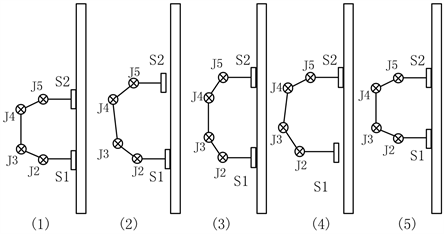Figure 11. Gait sketch of inchworm

1) 初始步态，尺蠖机器人两吸盘贴于目标平面。

2) 足S1保持吸附状态，足S2的吸盘脱附，关节J2、J3、J4和J5配合运动使足S2向上向前运动。

3) 关节J2、J3、J4和J5配合运动使足S2向下向前运动，等足S2运动至合适位置时，吸盘重新黏附到目标平面。

4) 足S2保持吸附状态，足S1的吸盘脱附，关节J2、J3、J4和J5配合运动使足S1向上向前运动。

5) 关节J2、J3、J4和J5配合运动使足S1向下向前运动，等足S1运动至合适位置时，吸盘重新黏附到目标平面。

${\theta }_{2}+{\theta }_{3}+{\theta }_{4}+{\theta }_{5}=-{180}^{\circ }$ (5-1)

${\theta }_{3}={\theta }_{4}$ (5-2)

5.2. 关节运动方程求解

5.2.1. 运动学方程的建立

${}^{0}A{}_{6}={}^{0}A{}_{1}{}^{1}A{}_{2}{}^{2}A{}_{3}{}^{3}A{}_{4}{}^{4}A{}_{5}{}^{5}A{}_{6}=\left[\begin{array}{cccc}{\stackrel{^}{x}}_{1}& {\stackrel{^}{y}}_{1}& {\stackrel{^}{z}}_{1}& {p}_{1}\\ {\stackrel{^}{x}}_{2}& {\stackrel{^}{y}}_{2}& {\stackrel{^}{z}}_{2}& {p}_{1}\\ {\stackrel{^}{x}}_{3}& {\stackrel{^}{y}}_{3}& {\stackrel{^}{z}}_{3}& {p}_{3}\\ 0& 0& 0& 1\end{array}\right]$ (5-3)

$\begin{array}{c}{}^{0}A{}_{6}={}^{0}A{}_{1}{}^{1}A{}_{2}{}^{2}A{}_{3}{}^{3}A{}_{4}{}^{4}A{}_{5}{}^{\text{5}}A{}_{\text{6}}\\ \text{=}\left[\begin{array}{cccc}{\stackrel{^}{x}}_{1}& {\stackrel{^}{y}}_{1}& {\stackrel{^}{z}}_{1}& {p}_{1}\\ {\stackrel{^}{x}}_{2}& {\stackrel{^}{y}}_{2}& {\stackrel{^}{z}}_{2}& {p}_{1}\\ {\stackrel{^}{x}}_{3}& {\stackrel{^}{y}}_{3}& {\stackrel{^}{z}}_{3}& {p}_{3}\\ 0& 0& 0& 1\end{array}\right]=\left[\begin{array}{cccc}1& 0& 0& {p}_{1}\\ 0& 1& 0& {p}_{1}\\ 0& 0& 1& {p}_{3}\\ 0& 0& 0& 1\end{array}\right]\end{array}$ (5-4)

${\theta }_{2}+{\theta }_{3}+{\theta }_{4}+{\theta }_{5}=-{180}^{\circ }$

$\left\{\begin{array}{l}{p}_{1}=0\\ {p}_{\text{2}}=-{a}_{1}-{a}_{2}\mathrm{cos}{\theta }_{2}-{a}_{3}\mathrm{cos}\left({\theta }_{2}+{\theta }_{3}\right)-{a}_{4}\mathrm{cos}\left({\theta }_{2}+{\theta }_{3}+{\theta }_{4}\right)+{a}_{5}\\ {p}_{\text{3}}=-{a}_{2}\mathrm{sin}{\theta }_{2}-{a}_{3}\mathrm{sin}\left({\theta }_{2}+{\theta }_{3}\right)-{a}_{4}\mathrm{sin}\left({\theta }_{2}+2{\theta }_{3}\right)\end{array}$ (5-5)

$\left\{\begin{array}{l}{p}_{1}=0\\ {p}_{2}=-7t\\ {p}_{3}={a}_{2}+{a}_{3}+7t\end{array}\text{ }\text{ }\text{ }t\in \left(0,3\text{\hspace{0.17em}}\text{s}\right)$ (5-6)

$\left\{\begin{array}{l}{p}_{1}=0\\ {p}_{2}=-21+7\left(t-3\right)\\ {p}_{3}={a}_{2}+{a}_{3}+21+7\left(t-3\right)\end{array}\text{ }\text{ }t\in \left(3\text{\hspace{0.17em}}\text{s},6\text{\hspace{0.17em}}\text{s}\right)$ (5-7)

$\left\{\begin{array}{l}{p}_{1}=0\\ {p}_{2}=7\left(t-6\right)\\ {p}_{3}={a}_{2}+{a}_{3}+42-7\left(t-6\right)\end{array}\text{ }\text{ }\text{ }t\in \left(6\text{\hspace{0.17em}}\text{s},9\text{\hspace{0.17em}}\text{s}\right)$ (5-8)

$\left\{\begin{array}{l}{p}_{1}=0\\ {p}_{2}=21-7\left(t-9\right)\\ {p}_{3}={a}_{2}+{a}_{3}+21-7\left(t-9\right)\end{array}\text{\hspace{0.17em}}\text{ }\text{ }\text{\hspace{0.17em}}\text{\hspace{0.17em}}t\in \left(9\text{\hspace{0.17em}}\text{s},12\text{\hspace{0.17em}}\text{s}\right)$ (5-9)

5.2.2. 运动学方程的求解

$t\in \left(0,3\text{\hspace{0.17em}}\text{s}\right)$ 时，由式(5-5)和(5-6)有：

$-7t=-{a}_{1}-{a}_{2}\mathrm{cos}{\theta }_{2}-{a}_{3}\mathrm{cos}\left({\theta }_{2}+{\theta }_{3}\right)-{a}_{4}\mathrm{cos}\left({\theta }_{2}+2{\theta }_{3}\right)+{a}_{5}$ (5-10)

${a}_{2}+{a}_{3}+7t=-{a}_{2}\mathrm{sin}{\theta }_{2}-{a}_{3}\mathrm{sin}\left({\theta }_{2}+{\theta }_{3}\right)-{a}_{4}\mathrm{sin}\left({\theta }_{2}+2{\theta }_{3}\right)$ (5-11)

$7t={a}_{2}\mathrm{cos}{\theta }_{2}+{a}_{3}\mathrm{cos}\left({\theta }_{2}+{\theta }_{3}\right)+{a}_{2}\mathrm{cos}\left({\theta }_{2}+2{\theta }_{3}\right)$ (5-12)

${a}_{2}+{a}_{3}+7t=-{a}_{2}\mathrm{sin}{\theta }_{2}-{a}_{3}\mathrm{sin}\left({\theta }_{2}+{\theta }_{3}\right)-{a}_{2}\mathrm{sin}\left({\theta }_{2}+2{\theta }_{3}\right)$ (5-13)

$4{a}_{2}^{2}{\mathrm{cos}}^{2}{\theta }_{3}+4{a}_{2}{a}_{3}\mathrm{cos}{\theta }_{3}+{a}_{3}^{2}-49{t}^{2}-{\left({a}_{2}+{a}_{3}+7t\right)}^{2}=0$ (5-14)

$\begin{array}{c}\mathrm{cos}{\theta }_{3}=\frac{-4{a}_{2}{a}_{3}±\sqrt{16{a}_{2}^{2}{a}_{3}^{2}-16{a}_{2}^{2}\left[{a}_{3}^{2}-49{t}^{2}-{\left({a}_{2}+{a}_{3}+7t\right)}^{2}\right]}}{8{a}_{2}{}^{2}}\\ =\frac{-{a}_{3}}{2{a}_{2}}±\frac{\sqrt{49{t}^{2}+{\left({a}_{2}+{a}_{3}+7t\right)}^{2}}}{2{a}_{2}}\end{array}$ (5-15)

${\theta }_{3}$ 有解，则需满足 $-1\le \mathrm{cos}{\theta }_{3}\le 1$ ，即：

$-1\le \frac{-{a}_{3}}{2{a}_{2}}+\frac{\sqrt{49{t}^{2}+{\left({a}_{2}+{a}_{3}+7t\right)}^{2}}}{2{a}_{2}}\le 1$

${\theta }_{3}=\mathrm{arccos}\left(\frac{-{a}_{3}+\sqrt{49{t}^{2}+{\left({a}_{2}+{a}_{3}+7t\right)}^{2}}}{2{a}_{2}}\right)={\theta }_{4}$ (5-16)

$\left(5\text{-}12\right)×\mathrm{cos}{\theta }_{2}$$\left(5\text{-13}\right)×\mathrm{sin}{\theta }_{2}$ 可得：

$7t\mathrm{cos}{\theta }_{2}={a}_{2}{\mathrm{cos}}^{2}{\theta }_{2}+{a}_{3}\mathrm{cos}{\theta }_{2}\mathrm{cos}\left({\theta }_{2}+{\theta }_{3}\right)+{a}_{4}\mathrm{cos}{\theta }_{2}\mathrm{cos}\left({\theta }_{2}+2{\theta }_{3}\right)$ (5-17)

$\left({a}_{2}+{a}_{3}+7t\right)sin{\theta }_{2}=-{a}_{2}{\mathrm{sin}}^{2}{\theta }_{2}-{a}_{3}sin{\theta }_{2}\mathrm{sin}\left({\theta }_{2}+{\theta }_{3}\right)-{a}_{2}sin{\theta }_{2}\mathrm{sin}\left({\theta }_{2}+2{\theta }_{3}\right)$ (5-18)

$7t\mathrm{cos}{\theta }_{2}-\left({a}_{2}+{a}_{3}+7t\right)sin{\theta }_{2}={a}_{2}+{a}_{3}\mathrm{cos}{\theta }_{3}+{a}_{2}\mathrm{cos}2{\theta }_{3}$ (5-19)

$\begin{array}{l}-\left({a}_{2}+{a}_{3}+7t\right)sin{\theta }_{2}+7t\mathrm{cos}{\theta }_{2}\\ ={a}_{2}+{a}_{3}\left(\frac{-{a}_{3}+\sqrt{49{t}^{2}+{\left({a}_{2}+{a}_{3}+7t\right)}^{2}}}{2{a}_{2}}\right)\\ \text{\hspace{0.17em}}\text{\hspace{0.17em}}+{a}_{2}\left[2{\left(\frac{-{a}_{3}+\sqrt{49{t}^{2}+{\left({a}_{2}+{a}_{3}+7t\right)}^{2}}}{2{a}_{2}}\right)}^{2}-1\right]\\ =\frac{49{t}^{2}+{\left({a}_{2}+{a}_{3}+7t\right)}^{2}-{a}_{3}\sqrt{49{t}^{2}+{\left({a}_{2}+{a}_{3}+7t\right)}^{2}}}{2{a}_{2}}\end{array}$ (5-20)

$\begin{array}{l}{k}_{1}=-\left({a}_{2}+{a}_{3}+7t\right)\\ {k}_{2}=10t\\ {k}_{3}=\frac{49{t}^{2}+{\left({a}_{2}+{a}_{3}+7t\right)}^{2}-{a}_{3}\sqrt{49{t}^{2}+{\left({a}_{2}+{a}_{3}+7t\right)}^{2}}}{2{a}_{2}}\end{array}$

${\theta }_{2}=\mathrm{arctan}2\left(\frac{{k}_{1}}{{k}_{2}}\right)±\mathrm{arctan}2\left(\frac{\sqrt{{k}_{1}^{2}+{k}_{2}^{2}-{k}_{3}^{2}}}{{k}_{3}}\right)$ (5-21)

${\theta }_{2}=\mathrm{arctan}2\left(\frac{{k}_{1}}{{k}_{2}}\right)+\mathrm{arctan}2\left(\frac{\sqrt{{k}_{1}^{2}+{k}_{2}^{2}-{k}_{3}^{2}}}{{k}_{3}}\right)$ (5-22)

$\begin{array}{l}{\theta }_{5}=\text{π}-{\theta }_{2}-{\theta }_{3}-{\theta }_{4}\\ \text{ }=\text{π}-\left[\mathrm{arctan}2\left(\frac{{k}_{1}}{{k}_{2}}\right)+\mathrm{arctan}2\left(\frac{\sqrt{{k}_{1}^{2}+{k}_{2}^{2}-{k}_{3}^{2}}}{{k}_{3}}\right)\right]\\ \text{ }\text{ }-2\mathrm{arccos}\left(\frac{-{a}_{3}+\sqrt{49{t}^{2}+{\left({a}_{2}+{a}_{3}+7t\right)}^{2}}}{2{a}_{2}}\right)\end{array}$ (5-23)

5.3. 虚拟样机建模及仿真

Adsms软件是一款常用的虚拟样机技术软件，可以用于运动学和动力学等的仿真。运用ADAMS软件对尺蠖机器人进行运动学仿真，可以验证运动学方程和步态规划结果的正确性。在软件中建立的模型如图12所示。

4个关节转角曲线图是相对于尺蠖机器人的初始位置的关节转角。从曲线图中可以看出，在运动过程中可以看出，四个关节的转角之和始终为0，考虑到初始位置有 ${\theta }_{2}+{\theta }_{3}+{\theta }_{4}+{\theta }_{5}=-{180}^{\circ }$ ，故运动过程中时刻满足 ${\theta }_{\text{2}}+{\theta }_{3}+{\theta }_{4}+{\theta }_{5}=-{180}^{\circ }$ ，且由动画可以看出尺蠖机器人的两个吸盘底部平面始终平行，与步态规划结果一致。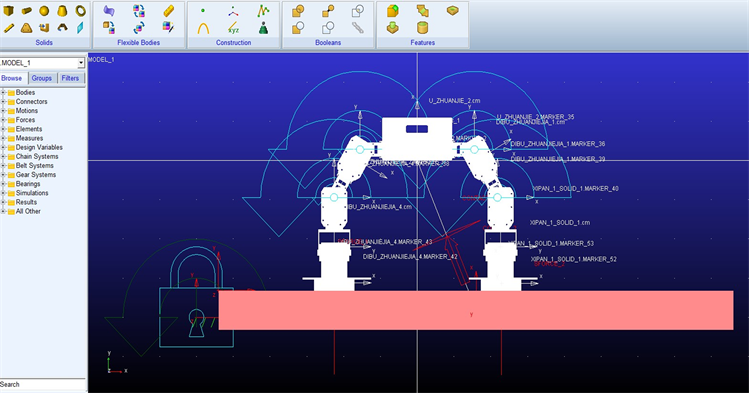Figure 12. The model of inchworm- like robot in Adams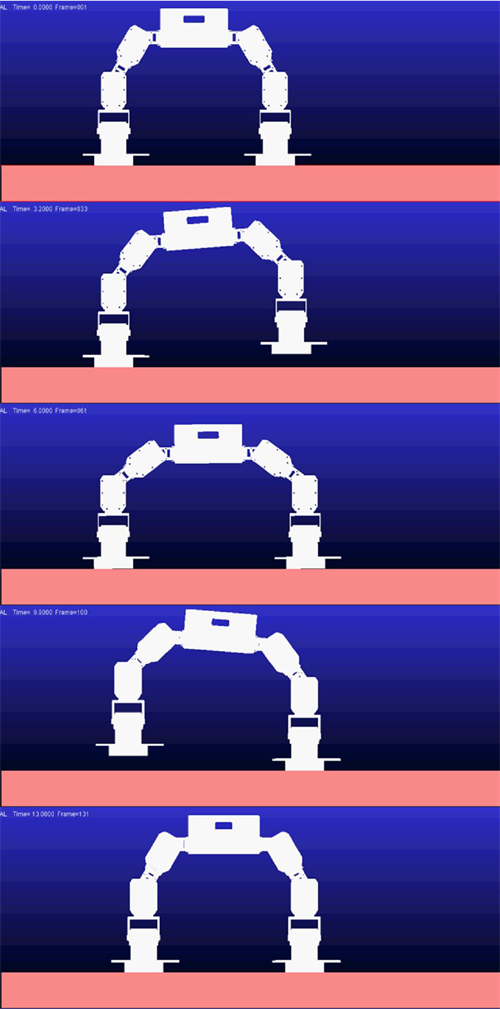Figure 13. The animation the robot’s inchworm gait in Adams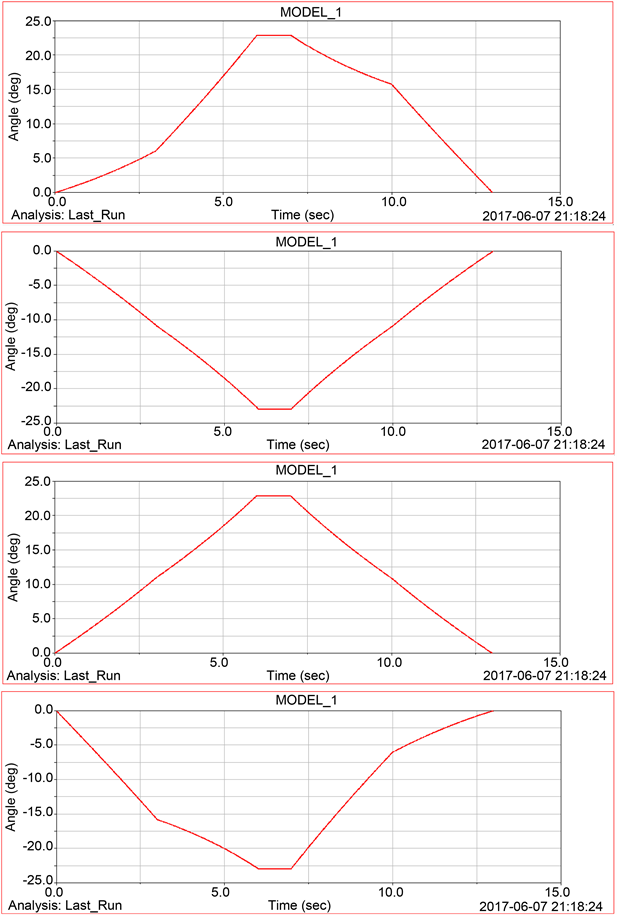Figure 14. Curve the joint 2,3,4,5’s rotation angle

6. 结论

The Design and Analysis of the Biomimetic Adherent Inchworm-Like Robot[J]. 国际航空航天科学, 2017, 05(04): 194-211. http://dx.doi.org/10.12677/JAST.2017.54022

1. 1. 李健, 葛本利. 浅析卫星在现代战争中的作用[J]. 数字通信世界, 2009(6): 52-55.

2. 2. 马继峰, 林金永, 高晓颖, 等. 空间攻防对抗体系的建模与仿真研究[J]. 系统仿真学报, 2007, 19(23): 5601-5602.

3. 3. 张俊华, 杨根, 徐青. 微小卫星的现状及其在空间攻防中的应用[J]. 航天电子对抗, 2008, 24(4): 16-17.

4. 4. Jeong, H.E., Lee, J.K., Kim, H.N., et a1. (2009) A Nontransferring Dry Adhesive with Hierarchicalpolymer nanohaks. Proceedings of the National Academy of Ciences, 106, 5639-5644. https://doi.org/10.1073/pnas.0900323106

5. 5. Geim, A.K., Dubonos, S.V., Grigorieva, I.V., et al. (2003) Microfabricated Adhesive Mimicking Gecko Foot-Hair. Nature Materials, 2, 464-463.

6. 6. Aksak, B., Murphy, M.P. and Sitti, M. (2007) Adhesion of Biologically Inspired Vertical and Angledpolymer Microfiber Arrays. Langrnuir, 23, 3322-3332. https://doi.org/10.1021/la062697t

7. 7. 戴振东, 彭福军. 空间机器人的研究与仿壁虎机器人关键技术[J]. 科学通报, 2015(32): 3114-3124.

8. 8. 龚玲. 碳纳米管阵列的转移及粘附性能研究[D]: [硕士学位论文]. 南京: 南京航空天大学, 2013: 17-56.

9. 9. 赵大伟. 双足机器人的步态规划与仿真研究[D]: [硕士学位论文]. 北京: 北京邮电大学, 2009: 11-21.

10. NOTES



*通讯作者。